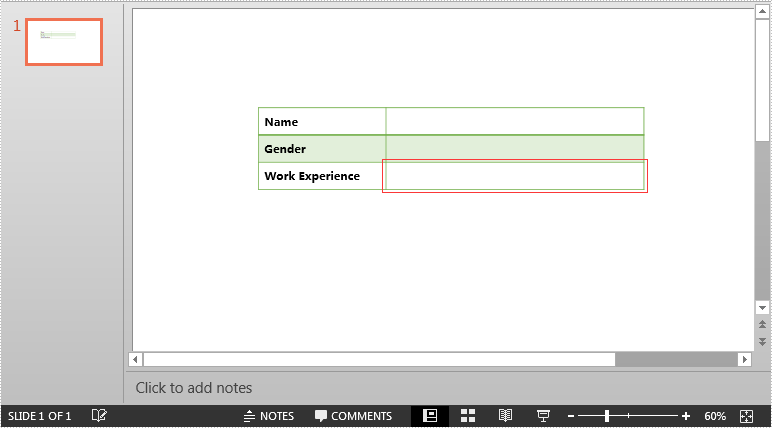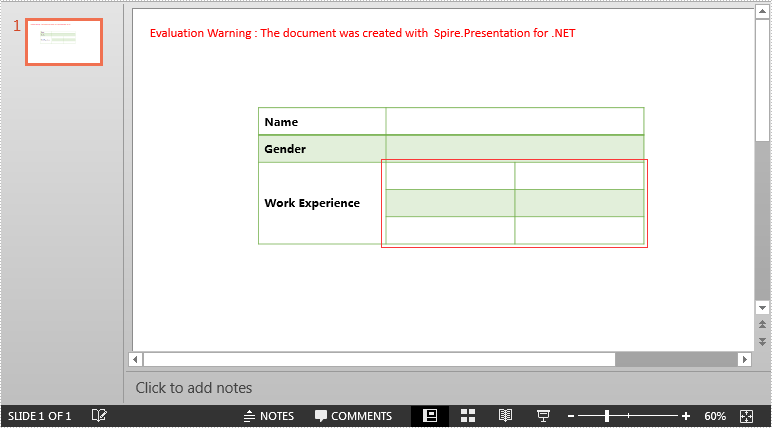# Split Table Cells in PowerPoint in C#

The original table:Detail steps:

Step 1: Instantiate a Presentation object and load the PowerPoint file.

```Presentation ppt = new Presentation();
```

Step 2: Get the first slide.

```ISlide slide = ppt.Slides;
```

Step 3: Get the table on the slide.

```ITable table = slide.Shapes as ITable;
```

Step 4: Split the cell [1, 2] into 3 rows and 2 columns.

```table[1, 2].Split(3, 2);
```

Step 5: Save the file.

```ppt.SaveToFile("Split.pptx", FileFormat.Pptx2013);
```

Screenshot after splitting:Full code:

```using Spire.Presentation;

namespace Split_Table_Cells_in_PPT
{
class Program
{
static void Main(string[] args)
{
//Instantiate a Presentation object
Presentation ppt = new Presentation();

//Get the first slide
ISlide slide = ppt.Slides;

//Get the table
ITable table = slide.Shapes as ITable;

//Split cell [1, 2] into 3 rows and 2 columns
table[1, 2].Split(3, 2);

//Save the file
ppt.SaveToFile("Split.pptx", FileFormat.Pptx2013);
}
}
}
```